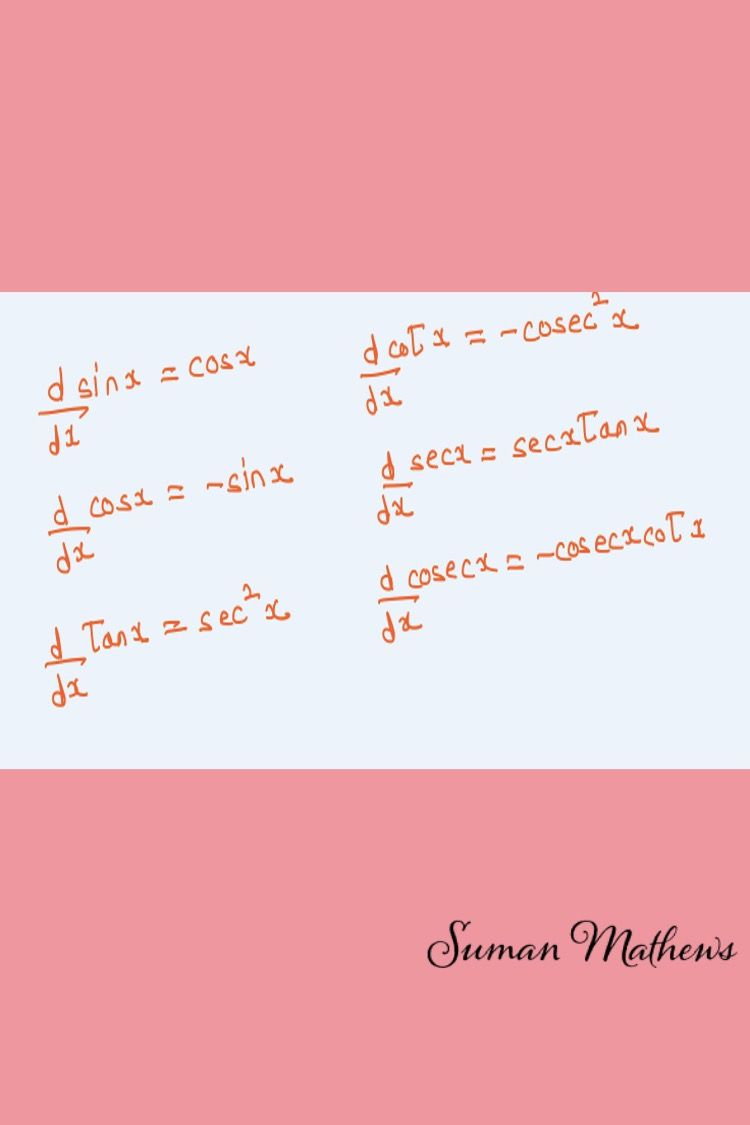top of page
Search

# 5 tips for getting a high score in calculus-class 11

Updated: May 19Limits and Derivatives form the basic foundation of Calculus. It's introduced in Class 11 briefly and followed up extensively in Class 12. Being a math educator teaching mathematics to high school and college students for over 3 decades, I can tell you what exactly you need to be learning in Limits and Derivatives for Class 11. All my students have found Limits and Derivatives incredibly easy after learning from me.

So, here are the best study tips for learning Calculus,- Class 11 Mathematics. When you understand these tips outlined here, you'll be able to solve any question on Limits and Derivatives with ease.

## Tip #1 - Understand the basic definition of a limit and how to apply it in problem solving

As you come across the definition of a limit for the first time, it says that as x approaches a neighbourhood of a, the function approaches a neighbourhood of l. This may seem a little difficult to comprehend for the first time.

But as I tell my students, when it comes to problem solving, it's as simple as just substituting the value of x, provided the denominator is not zero.

What happens if the denominator is zero? then we work around the function so that the denominator does not tend to zero. Again there are exceptions, but this is the basic rule to follow.

## Tip #2 - Learn results on the basic algebra of limits and some important theorems on limits

There are some basic results such as limit of a sum is equal to the sum of limits, limit of a difference is equal to the difference of limits, limit of a product /quotient is equal to the product/quotient of limits. My students find this incredibly easy.

There are also some basic theorems on algebraic limits. What's most important is that you understand the concept of left hand and right hand limits and how to use it in problem solving.

"Knowing limits is essential to understanding Calculus."## Tip #3 - Progress to Trigonometric Limits

Next you will learn about trigonometric limits. There are a couple of formulas used here. The formula of cos2x is used extensively here. As I tell my students, this formula is used practically in every Calculus class. While problem solving, you end up using all the trigonometric formulas that you have learnt.

## Tip #4 - Limits of exponential and logarithmic functions and introduction to Derivatives

There are limits of exponential and algebraic functions which are very important as they are used in Class 12 in Indeterminate form of Limits. I have had students using these formulas in college mathematics as well

From here, you will be introduced to derivatives. The very basic definition of a derivative starts with limits. That's precisely why you learn limits first and then derivatives. My students are not particularly fond of evaluating derivatives using the definition. They feel it's much more easier to use the formula and evaluate the derivatives.

But then, as I keep telling them, learning how to use the definition for calculating the derivative is necessary. Moving on, you will come to the formula for calculating derivatives. You need to know certain basic formulas such as algebraic formulas, derivative of a constant and most important derivatives of trigonometric functions.

There is also the product rule and the Quotient rule. My students are able to manage the product rule easily but when it comes to the quotient rule, they get confused initially. But then, with practice they are able to overcome it.

You are also just introduced to the chain rule in Class 11, but then that is just a tip of the iceberg and you'll use it later in Class 12.## Tip #5 - How to get a high score in Calculus Class 11

Just reading this will go a long way in helping you understand Limits and Derivatives in Calculus. I have provided some useful formulas which will go a long way in your study. My students just love it when I give them the formulas readymade. I hope you like it too! To learn more, visitYou have understood how to go about studying Limits and Derivatives but still need that extra help. You can contact me for online tutoring. I have been teaching High School and College Mathematics for over 3 decades. Most of my students have secured above 90% in Mathematics and quite a few have scored a 100.

Quite a few of them have chosen to pursue Math as their further study. I tutor online and in groups of 5 to 10. You can avail the most convenient option for you. When you join my classes, you'll see your marks rising steadily!

But wait----. The most important takeaway is that you need to practice math on a daily basis to excel in it.. Once you excel in Math, the sky is your limit!

Would you care to spread this blog around to students who need that little extra attention in Math. I also offer a referral fee for referring students. Again T&C apply.

I would love to hear from you.